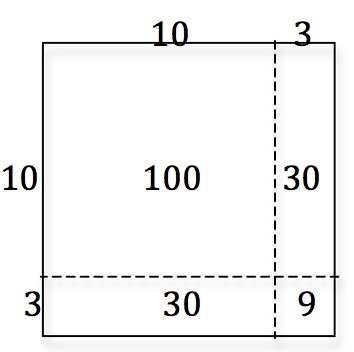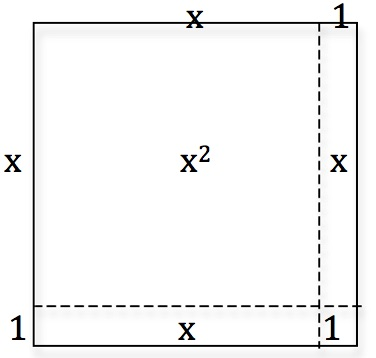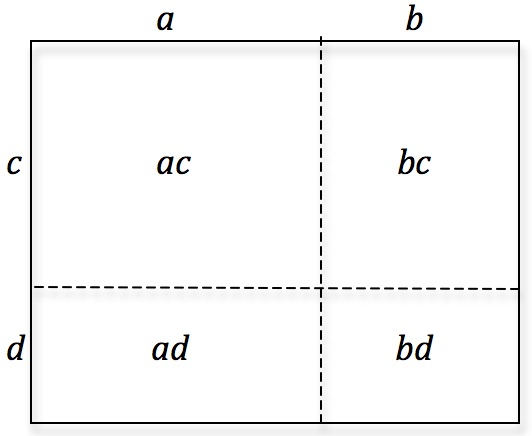Explain how the diagrams show how to work out 13 times 13 and (x+1) times (x+1).

Draw similar diagrams for 15 times 15 and (x+2) times (x+2).

Draw your own diagrams and use the same method to find the algebraic expressions for $$(x + 3)^2$$ and $$(2x + 5)^2$$ and work out the expansion of $$(a + b)(c + d)$$.Tagged with: